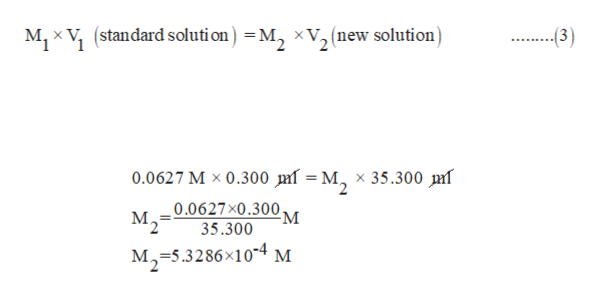# Calculate the concentration of an unknown solution that produced a current of 4.78 microA. The current went up to 6.54 microA after 35 mL of the unknown was added to 0.300 mL of 0.0627 M standard solution.

Question
54 views

Calculate the concentration of an unknown solution that produced a current of 4.78 microA. The current went up to 6.54 microA after 35 mL of the unknown was added to 0.300 mL of 0.0627 M standard solution.

check_circle

Step 1

As given that unknown solution produced a current of 4.78 micro A.

Since there is a relationship between current and concentration is expressed by equation (1) where I is limiting current and C is the concentration of solution and k is limiting current constant

Substitutes 4.78 micro A for I, in equation 1

Since 1 micro A= 1 × 10-6 A. So

Step 2

Also given that concentration of the standard solution is 0.0627 M  and when 35 ml of unknown solution is added to 0.300 ml of the standard solution than current reaches 6.54 × 10-6 A.

Using equation (3) we find the new concentration of the solution in which standard and the unknown solution are mixed.

In equation 3 M1 is the concentr...help_outlineImage TranscriptioncloseM, x V (standard soluti on)M, xV, (new solution) (3) 0.0627 M x 0.300 mf = M, x 35.300 mf 0.0627x0.300 -м M2 35.300 M2-5.3286x104 M fullscreen

### Want to see the full answer?

See Solution

#### Want to see this answer and more?

Solutions are written by subject experts who are available 24/7. Questions are typically answered within 1 hour.*

See Solution
*Response times may vary by subject and question.
Tagged in

### Electrochemistry